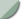#Outputting XML scoretables for display in Flash

If the Flash game has built in Hi-score display (such as this one), compared with an external HTML Hi-score display (such as this example), then there needs to be a method of getting the scores to Flash in a way it understands. The best way to do this is with XML. A server-side script is needed to putput this in the correct format when called, and the games from Galaxy Graphics follow one of two methods for the required XML format.

### XML Scores Type A

Where the score follows the name, with one node per item. Sample XML is below

<?xml version="1.0" encoding="utf-8"?>
<scoretable>
<name>John</name>
<score>58713</score>
<name>Carl</name>
<score>69311</score>
<name>Bob</name>
<score>72670</score>
</scoretable>

The PHP code snippet that generates this is as follows:

echo "<?xml version=\"1.0\" encoding=\"utf-8\"?>\n";
echo "<scoretable>\n";
while(\$myrow=mysql_fetch_array(\$result))
{
echo "<name>".htmlspecialchars(trim(\$myrow["name"]))."</name>\n";
echo "<score>".htmlspecialchars(trim(\$myrow["score"]))."</score>\n";
}
echo "</scoretable>";

Note this code already assumed you've connected to your database and run an SQL Query to list the scores in order.

### XML Scores Type B

Where the score and name are in the same node, with the name as an attribute of the score. Sample XML is below.

<?xml version="1.0" encoding="utf-8"?>
<scoretable>
<score xname="John">35478</score>
<score xname="Carl">38721</score>
<score xname="Bob">40161</score>
</scoretable>

The PHP code snippet that generates this is as follows:

echo "<?xml version=\"1.0\" encoding=\"utf-8\"?>\n";
echo "<scoretable>\n";
while(\$myrow=mysql_fetch_array(\$result))
{
echo "<score xname=\"".htmlspecialchars(trim(\$myrow["name"]))
."\">".htmlspecialchars(trim(\$myrow["score"]))."</score>\n";
}
echo "</scoretable>";

Note this code already assumed you've connected to your database and run an SQL Query to list the scores in order.Site content Copyright © 2001-2014 Galaxy Graphics Limited. | Privacy Policy | Terms of use